# Introduction of the Composed Mode of Exams— Part Mode¶

When we organize an exam, we need to choose the composed mode of the exam before adding test questions. We can choose Pre-Selected (i.e. fixed test questions), Random questions, or Pre+ Random mode (i.e. fixed + random mode), but in fact, many users have not yet used a very useful exam composed mode-[Compose Exams by Part].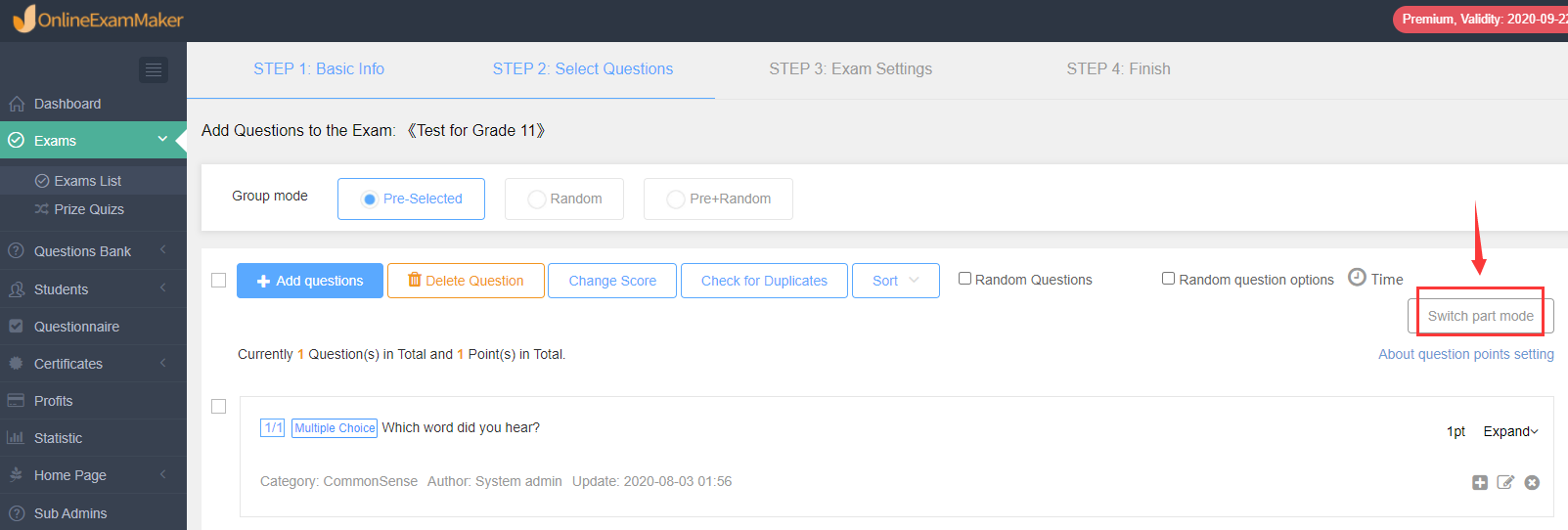As shown in the picture above, have you noticed the button of [Switch Part Mode]? What is the purpose of this exam composed function? Let’s take a look together.

#### What are the advantages of composing exams by part ?¶

The way the questions are partly composed is closer to the structure of the school’s exams. Usually, each subject has different question types, such as multiple choice questions, true or false questions, and cloze questions.

Therefore, the advantage of [Compose Exams by Part] is to help schools, education and training institutions simulate offline exams, and to display each module’s question types more clearly.

Let us take a look at how to use this feature.

#### Case Simulation of Composing Exams by Part¶

(1) [Switch Part Mode]

When we enter the second step of creating exams, and after adding a test question, click [Switch Part Mode] on the right to make questions by part.Below we simulate a set of practice questions:

(2) [Compose Exams by Part]

First add section 1 as multiple-choice questions, you can add a description for it, for example, you can list the number of questions in this section, the total number of points, and the answering rules.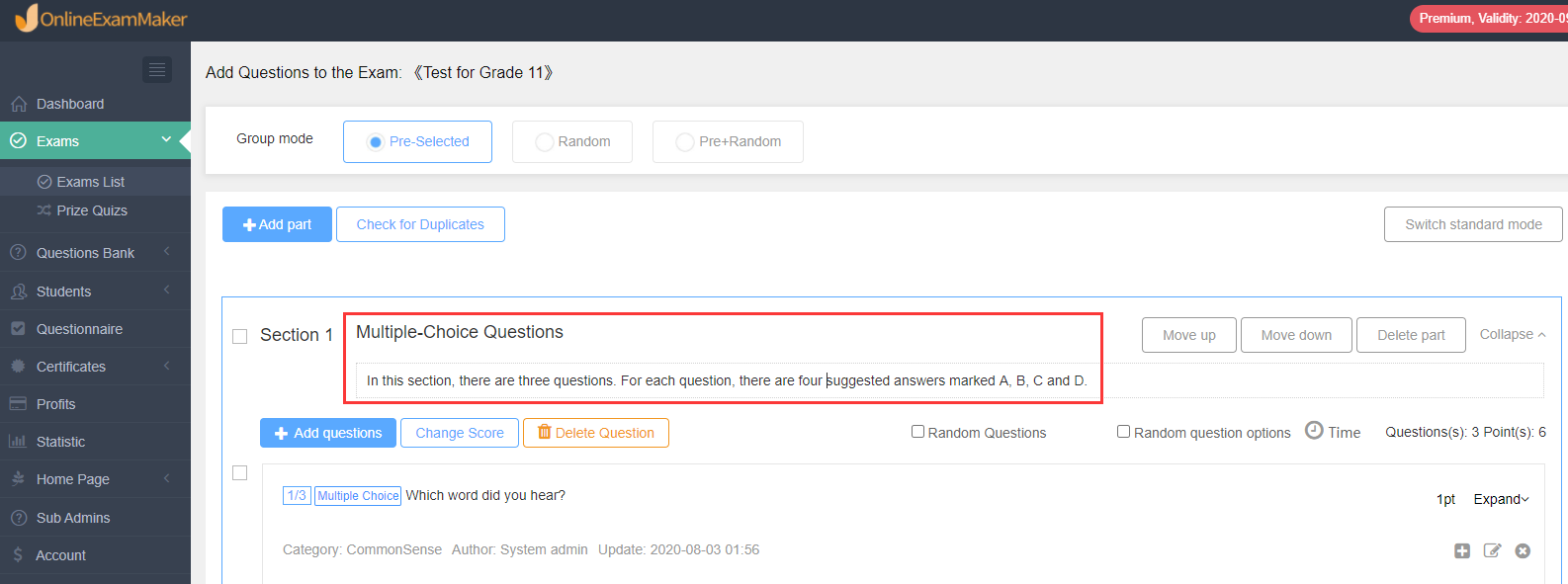After adding the description of this section, you can start adding questions.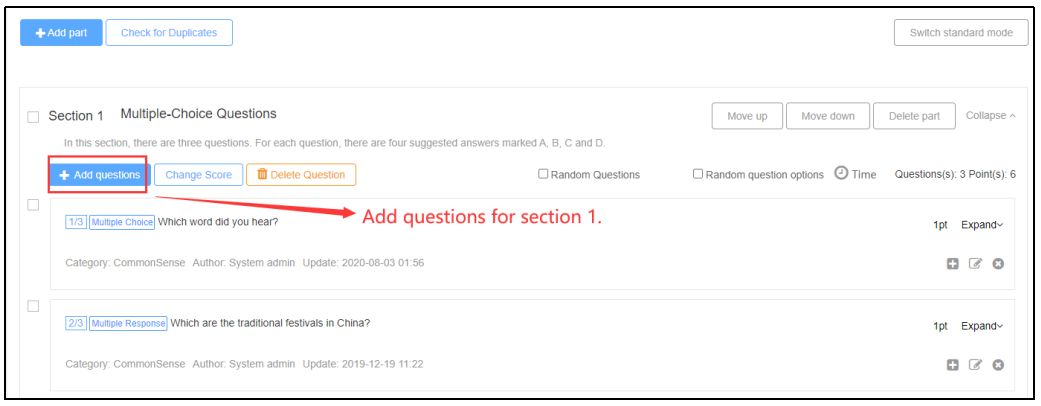Then, click [Add Part] at the top left to add a second section.As shown in the figure, add the second part as true or false questions, and add a description of this section and add the corresponding test questions.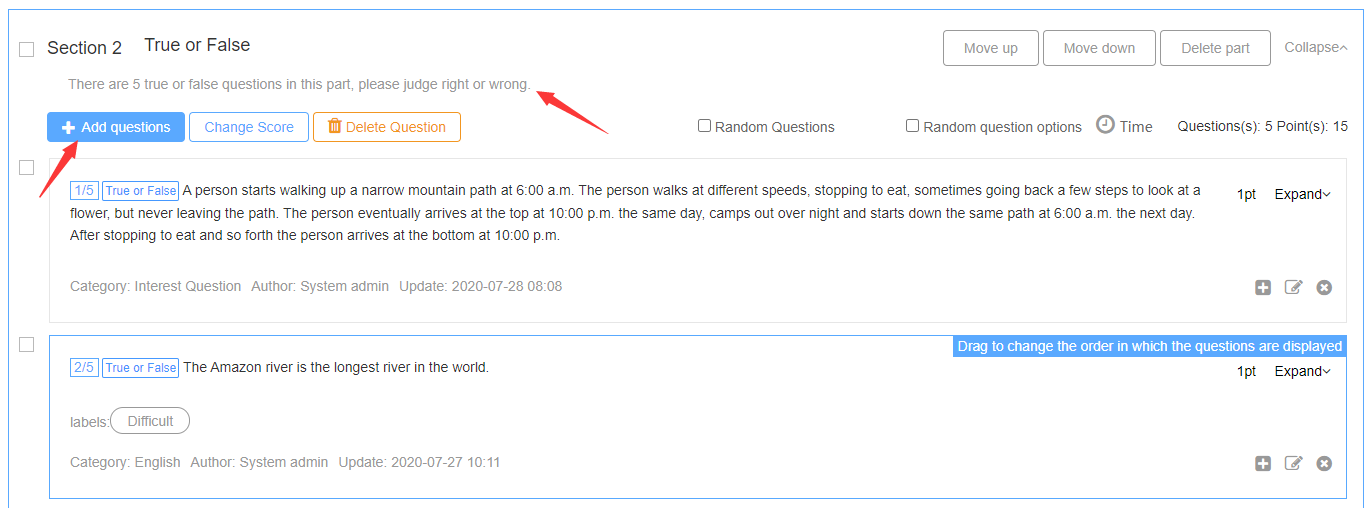Finally, also click [Add Part] to add a third section, as shown in the figure, add the third section as cloze questions, add a description of this section and add the corresponding test questions.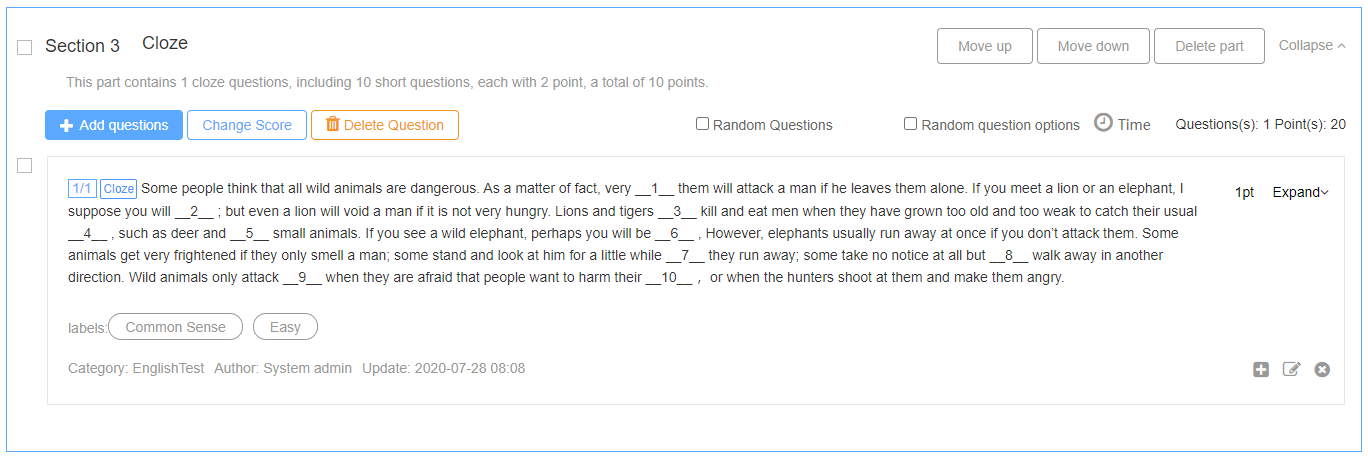After the test questions are added, set the test scores, and then set the parameters to publish the test papers.

#### How can candidates enter the exam?¶

Candidates can enter the exam as usual. The difference is that the system will display each part, and each part will display the number of questions, total score, and description;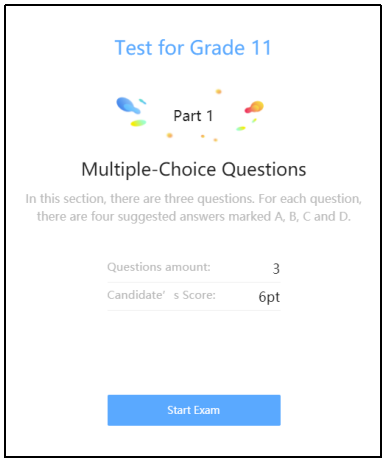When we finish the first part, we can continue to answer the questions in the second part according to the instructions.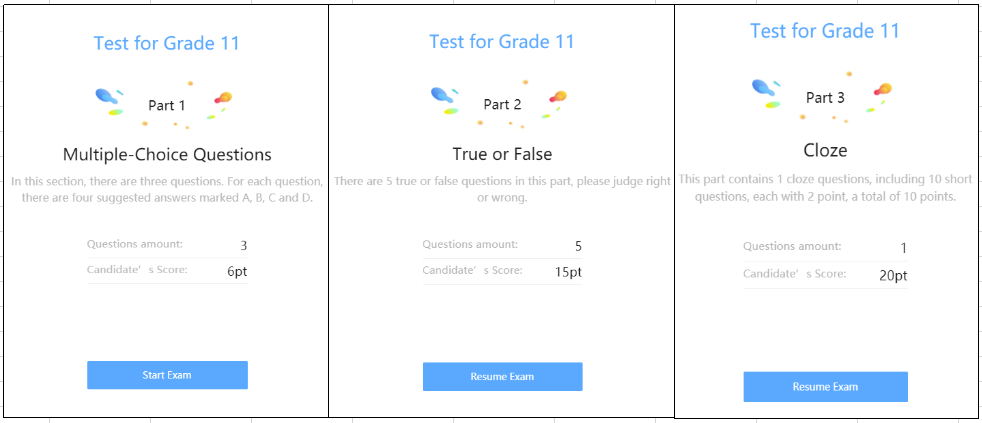When answering questions on the computer, candidates can clearly see the title of each part and the corresponding question number in the position of the answer sheet.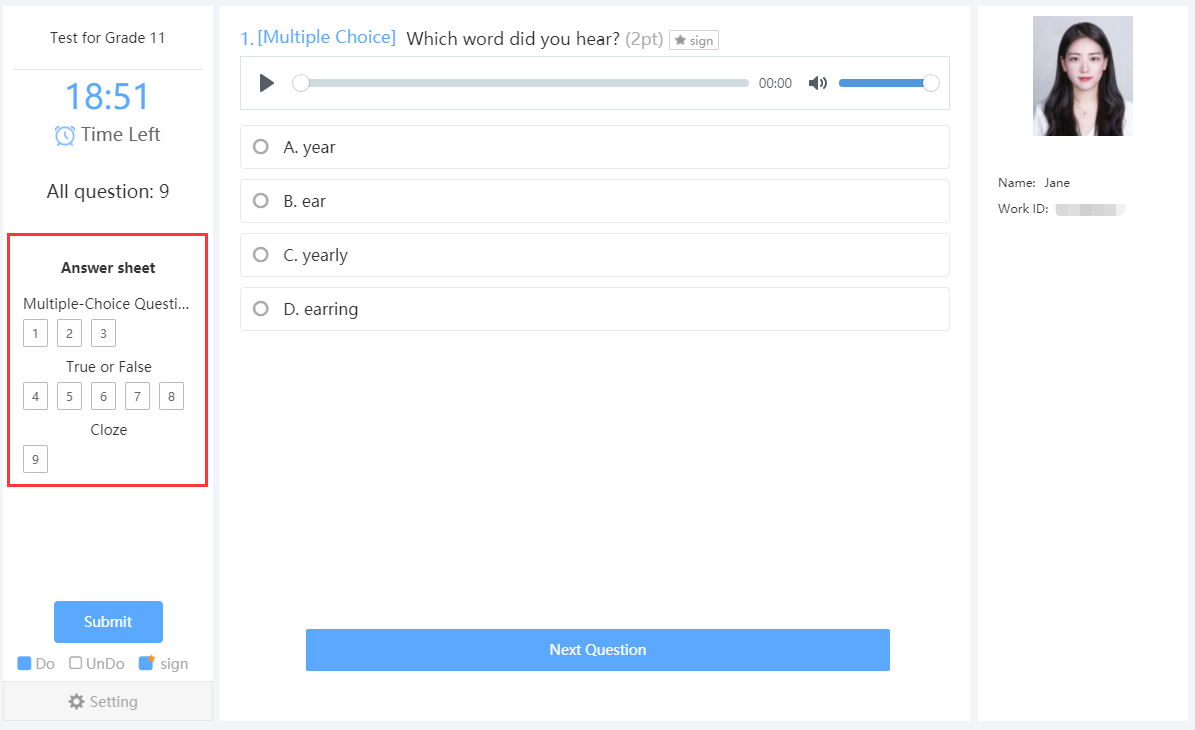Scan the QR code below to experience the feature online